In :
import numpy as np
import time
import torch
import matplotlib.pyplot as plt
%matplotlib inline


# Very Brief Introduction to Autograd¶

Let's use torch.autograd to calculate the derivative of the sine function. Here is what we should get.

In :
x = np.linspace(-2*np.pi, 2*np.pi, 100)
y = np.sin(x)
dy = np.cos(x)
plt.plot(x, y)
plt.plot(x, dy)
plt.legend(('$\sin(x)$', '$\\frac{d \sin(x)}{dx} = \cos(x)$'));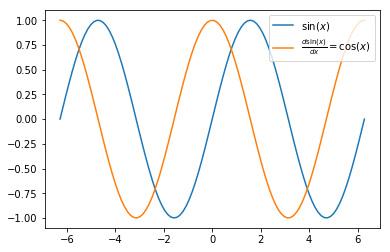The autograd module in pytorch is designed to calculate gradients of scalar-valued functions with respect to parameters. So, how can we use autograd to calculate $\frac{d \sin(x)}{dx}$ for multiple values of $x$? Well, we can't with a single call to backward. Instead, we must include a vector as an argument to backward that has as many elements as $x$ has. The dot product of the vector and the calculated gradient is calculated, to sum up the full gradient with respect to each component in the vector. This is just what is needed when calculating a gradient of a model's mean squared error, averaged over all outputs, with respect to the model's parameters.

Back to our original problem. We can calculate the derivative of $\sin(x)$ with respect to each value of $x$ by calling backward once for each value of $x$ and setting the vector argument to backward to all zeros except one value corresponding to the position of the value of $x$ we want the derivative for.

The following bit of code illustrates this. Sharif first showed this to me. This site also helps.

In :
x = torch.autograd.Variable(torch.linspace(-2*np.pi, 2*np.pi, steps=100), requires_grad=True)


The variable x will contain the gradient of y (defined in next cell) with respect to x, but only after y.backward(...) is called. Any additional calls to y.backward(...) will add gradient values to the current gradient values. The following test and call to x.grad.data.zero_() will zero the gradient values, to take care of the case when then next three cells are executed additional times.

In :
if x.grad is not None:

y = torch.sin(x)

In :
dout = torch.zeros(100)
for i in range(100):
dout[:] = 0
dout[i] = 1
y.backward(dout, retain_graph=True)

In :
plt.plot(x.data.numpy(), y.data.numpy());
plt.legend(('$\sin(x)$', '$\\frac{d \sin(x)}{dx} = \cos(x)$'));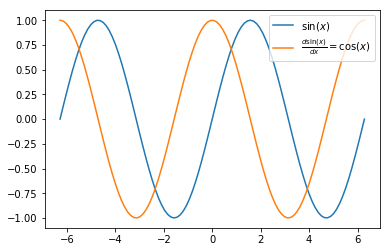# Using Numpy to Fit a Polynomial to Data¶

Let's try to fit a polynomial to the sine function. First, here is the parameterized polynomial model of degree 5 and its derivative.

In :
def poly(x, w):
''' poly(x,w), where x is Nx1 samples and w is 1xD+1 coefficients for x^0, x^1, ..., x^D'''
D = w.size
xPowers = x ** range(D)
return xPowers @ w


The derivative of a polynomial of degree 3 is

$$\frac{d (w_0 + w_1 x + w_2 x^2 + w_3 x^3}{dw_i} = (1, x, x^2, x^3)$$

and in python it is

In :
def dpoly_dw(x, w):
D = w.size
xPowers = x ** range(D)
return xPowers


Let's test these functions.

In :
x = np.linspace(-5, 5, 20).reshape((-1,1))
w = 0.1*np.array([3.0, -2.0, -1.5, 5]).reshape((-1,1))
x.shape, w.shape

Out:
((20, 1), (4, 1))
In :
poly(x, w).shape, dpoly_dw(x,w).shape

Out:
((20, 1), (20, 4))
In :
plt.subplot(2, 1, 1)
plt.plot(poly(x, w))
plt.ylabel('poly(x,w)')
plt.subplot(2,1,2)
plt.plot(dpoly_dw(x,w))
plt.ylabel('d poly(x,w)/ dw');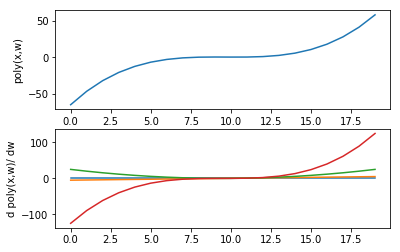Now, some data to fit. 100 samples of part of the sine curve.

In :
x = np.linspace(0, 5, 100).reshape((-1, 1))
y = np.sin(x)
plt.plot(x, y, 'o-');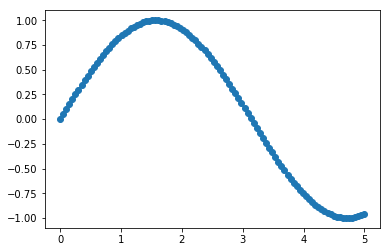Okay, ready to fit the polynomial to this data. Steps are simple. Initialize w to zeros. Calculate output of polynomial model. Update w by negative gradient of mean squared error with respect to w, multiplied by a small learning rate. To make plot of mse versus number of update steps, calculate mean squared error between model output and data.

In :
startTime = time.time()

nSteps = 200000
learnRate = 0.00001
degree = 4

w = np.zeros((degree+1, 1))
mseTrace = np.zeros(nSteps)
nSamples = x.shape

for step in range(nSteps):

yModel = poly(x, w)
grad = -2/nSamples * dpoly_dw(x, w).T @ (y - yModel)

if step == 0:

mse = ((y - yModel)**2).mean()
mseTrace[step] = mse

print('Numpy took {} seconds'.format(time.time()-startTime))

First step gradient:
[[  -0.27402023]
[   0.98929269]
[   7.41287669]
[  38.07474351]
[ 180.01689072]]
Numpy took 13.437073707580566 seconds

In :
plt.figure(figsize=(15, 5))
plt.subplot(1, 2, 1)
plt.plot(mseTrace)

plt.subplot(1, 2, 2)
plt.plot(x, y)
plt.plot(x, yModel);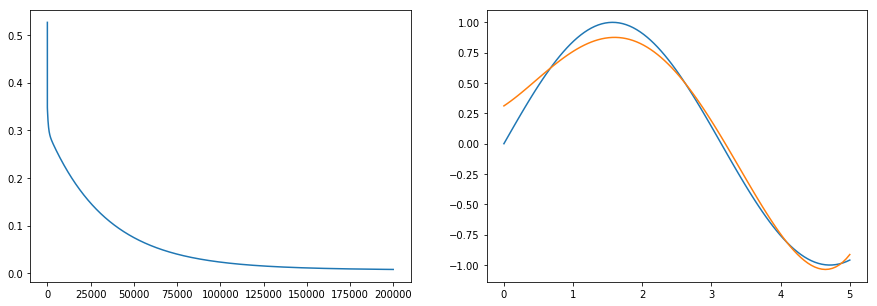# Now, with Pytorch¶

Now we repeat all of the above function definitions with changes needed for implementation in torch instead of numpy.

In :
dtype = torch.FloatTensor

In :
def poly_torch(x, w):
''' poly(x,w), where x is Nx1 samples and w is 1xD+1 coefficients for x^0, x^1, ..., x^D'''
# D = w.size
D = w.shape
xPowers = x ** torch.arange(0.0, D)
# return xPowers @ w
return xPowers.mm(w)

# No changes needed from dpoly

def dpoly_dw_torch(x, w):
D = w.shape
xPowers = x ** torch.arange(0.0, D)
return xPowers

In :
x_torch = torch.from_numpy(x).type(dtype)
y_torch = torch.from_numpy(y).type(dtype)

In :
startTime = time.time()

nSteps = 200000
learnRate = 0.00001
degree = 4

# w = np.zeros((degree+1, 1))
w_torch = torch.zeros((degree+1, 1)).type(dtype)

# mseTrace = np.zeros(nSteps)
mseTrace = torch.zeros(nSteps)
nSamples = x_torch.shape

for step in range(nSteps):

# yModel = poly(x, w)
# grad = -2/nSamples * dpoly(x, w).T @ (y - yModel)
yModel = poly_torch(x_torch, w_torch)
grad = -2/nSamples * dpoly_dw_torch(x_torch, w_torch).t().mm(y_torch - yModel)

if step == 0:

mse = ((y_torch - yModel)**2).mean()
mseTrace[step] = mse

print('Pytorch took {} seconds'.format(time.time()-startTime))

First step gradient:

-0.2740
0.9893
7.4129
38.0747
180.0169
[torch.FloatTensor of size 5x1]

Pytorch took 11.846007585525513 seconds

In :
plt.figure(figsize=(15,5))
plt.subplot(1,2,1)
plt.plot(mseTrace.numpy())

plt.subplot(1,2,2)
plt.plot(x, y)
plt.plot(x, yModel.numpy());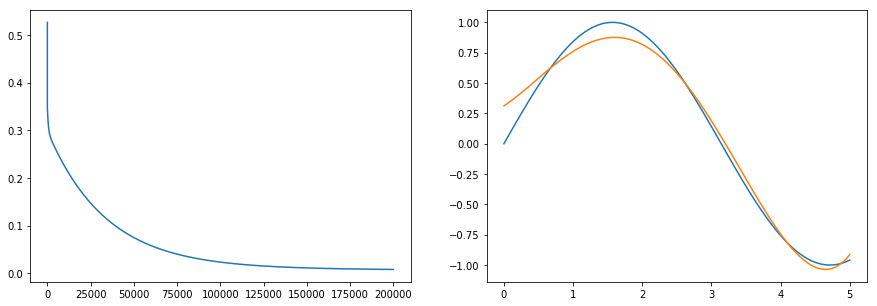Now let's remove the call to dpoly_dw_torch and use autograd to calculate the gradient of the polynomial with respect to w.

In :
def poly_torch(x, w):
''' poly(x,w), where x is Nx1 samples and w is 1xD+1 coefficients for x^0, x^1, ..., x^D'''
# D = w.size
# D = w.shape
D = w.data.shape
xPowers = x ** torch.autograd.Variable(torch.arange(0.0, D))
# return xPowers @ w
return xPowers.mm(w)

In :
startTime = time.time()

nSteps = 200000
learnRate = 0.00001
degree = 4

# w = np.zeros((degree+1, 1))
# w_torch = torch.zeros((degree+1, 1)).type(dtype)

# mseTrace = np.zeros(nSteps)
mseTrace = torch.zeros(nSteps)
nSamples = x_torch_Var.data.shape

for step in range(nSteps):

# yModel = poly(x, w)
# grad = -2/nSamples * dpoly(x, w).T @ (y - yModel)
yModel = poly_torch(x_torch_Var, w_torch_Var)
mse = ((y_torch_Var - yModel)**2).mean()

if step > 0:
mse.backward()

# grad = -2/nSamples * dpoly_torch(x_torch, w_torch).t().mm(y_torch - yModel)

if step == 0:

mseTrace[step] = mse.data

print('Pytorch with autograd took {} seconds'.format(time.time()-startTime))

  -0.2740
0.9893
7.4129
38.0747
180.0169
[torch.FloatTensor of size 5x1]

Pytorch with autograd took 44.704875230789185 seconds

In :
plt.figure(figsize=(15,5))
plt.subplot(1,2,1)
plt.plot(mseTrace.numpy())

plt.subplot(1,2,2)
plt.plot(x, y)
plt.plot(x, yModel.data.numpy());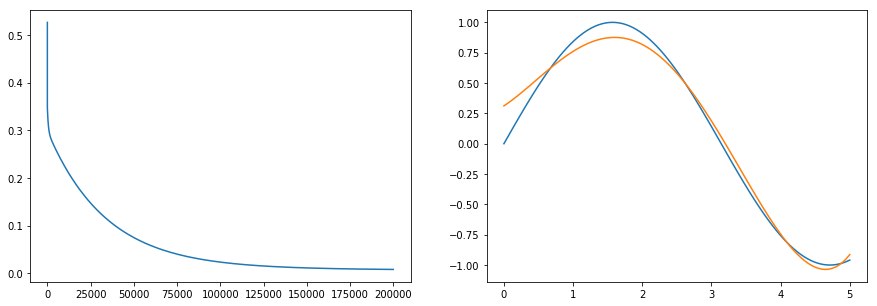# Pytorch with autograd on GPU¶

To run our torch implementation on the GPU, we need to change the data type and also call cpu() on variables to move them back to the CPU when needed.

First, here are the details of the GPU on this machine.

In :
!nvidia-smi

Tue Oct 10 15:35:55 2017
+-----------------------------------------------------------------------------+
| NVIDIA-SMI 384.59                 Driver Version: 384.59                    |
|-------------------------------+----------------------+----------------------+
| GPU  Name        Persistence-M| Bus-Id        Disp.A | Volatile Uncorr. ECC |
| Fan  Temp  Perf  Pwr:Usage/Cap|         Memory-Usage | GPU-Util  Compute M. |
|===============================+======================+======================|
|   0  NVS 315             Off  | 00000000:02:00.0 N/A |                  N/A |
| 30%   45C    P8    N/A /  N/A |    104MiB /   964MiB |     N/A      Default |
+-------------------------------+----------------------+----------------------+
|   1  GeForce GTX TIT...  Off  | 00000000:03:00.0 Off |                  N/A |
| 22%   43C    P8    16W / 250W |     12MiB / 12207MiB |      0%      Default |
+-------------------------------+----------------------+----------------------+

+-----------------------------------------------------------------------------+
| Processes:                                                       GPU Memory |
|  GPU       PID  Type  Process name                               Usage      |
|=============================================================================|
|    0                  Not Supported                                         |
+-----------------------------------------------------------------------------+

In :
!uptime

 15:35:55 up 10 days, 21:34,  1 user,  load average: 0.84, 0.40, 0.33

In :
dtype = torch.cuda.FloatTensor

In :
def poly_torch(x, w):
''' poly(x,w), where x is Nx1 samples and w is 1xD+1 coefficients for x^0, x^1, ..., x^D'''
# D = w.size
# D = w.shape
dtype = x.data.type()
D = w.data.shape
xPowers = x ** torch.autograd.Variable(torch.arange(0.0, D).type(type(x.data))) # .type(dtype))
# return xPowers @ w
return xPowers.mm(w)

In :
startTime = time.time()

nSteps = 200000
learnRate = 0.00001
degree = 4

# w = np.zeros((degree+1, 1))
# w_torch = torch.zeros((degree+1, 1)).type(dtype)

# mseTrace = np.zeros(nSteps)
mseTrace = torch.zeros(nSteps).type(torch.cuda.FloatTensor)
nSamples = x_torch_Var.data.shape

for step in range(nSteps):

# yModel = poly(x, w)
# grad = -2/nSamples * dpoly(x, w).T @ (y - yModel)
yModel = poly_torch(x_torch_Var, w_torch_Var)
mse = ((y_torch_Var - yModel)**2).mean()

if step > 0:
mse.backward()

# grad = -2/nSamples * dpoly_torch(x_torch, w_torch).t().mm(y_torch - yModel)

if step == 0:

mseTrace[step] = mse.data

print('Pytorch with autograd on GPU took {} seconds'.format(time.time()-startTime))

  -0.2740
0.9893
7.4129
38.0747
180.0169
[torch.cuda.FloatTensor of size 5x1 (GPU 0)]

Pytorch with autograd on GPU took 243.74856853485107 seconds

In :
plt.figure(figsize=(15,5))
plt.subplot(1,2,1)
plt.plot(mseTrace.cpu().numpy())

plt.subplot(1,2,2)
plt.plot(x, y)
plt.plot(x, yModel.data.cpu().numpy());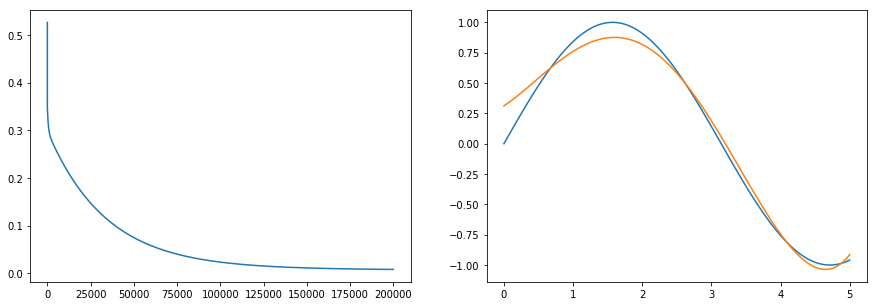# Wrapped up in one function¶

We can use the type of the data passed into these functions to select code appropriate for use with numpy.ndarray, torch.FloatTensor, or torch.autograd.variable.Variable.

In :
def poly(x, w):
''' poly(x,w), where x is Nx1 samples and w is 1xD+1 coefficients for x^0, x^1, ..., x^D'''
typex = type(x)

D = w.data.shape

elif typex is torch.FloatTensor or typex is torch.cuda.FloatTensor:
D = w.shape
exponents = torch.arange(0.0, D).type(typex)

else: # numpy
D = w.shape
exponents = np.arange(D, dtype=x.dtype)

xPowers = x ** exponents

if typex is np.ndarray:
return xPowers @ w
else:
return xPowers.mm(w)

def dpoly_dw(x, w):
typex = type(x)

D = w.data.shape

elif typex is torch.FloatTensor or typex is torch.cuda.FloatTensor:
D = w.shape
exponents = torch.arange(0.0, D).type(typex)

else: # numpy
D = w.shape
exponents = np.arange(D,dtype=x.dtype)

return x ** exponents

In :
def train(x, y, nSteps=200000, learnRate=0.00001, degree=4, use_torch=False, use_autograd=False, use_gpu=False):
startTime = time.time()

nSamples = x.shape

# Make sure use_torch is true if either of use_autograd or use_gpu is true
if use_gpu:
use_torch = True
use_torch = True

# initialize weights to be all zeros
w = np.zeros((degree+1, 1), dtype=np.float32)
if use_torch:
w = torch.from_numpy(w)
if use_gpu:
w = w.type(torch.cuda.FloatTensor)

# Change type of input samples, x, and targets, y
if use_torch:
x = torch.from_numpy(x).type(torch.FloatTensor)
y = torch.from_numpy(y).type(torch.FloatTensor)
if use_gpu:
x = x.type(torch.cuda.FloatTensor)
y = y.type(torch.cuda.FloatTensor)

# Set up array to store trace of MSE values for plotting later
mseTrace = np.zeros(nSteps, dtype=np.float32)
if use_torch:
mseTrace = torch.from_numpy(mseTrace)
if use_gpu:
mseTrace = mseTrace.type(torch.cuda.FloatTensor)

# Train for nSteps passes through data set
for step in range(nSteps):

# Forward pass through model, for all samples in x
yModel = poly(x, w)  # poly uses type of x to figure out what to do

# MSE, necessary for autograd. For all, needed for mseTrace.
mse = ((y - yModel)**2).mean()

# Backward pass to calculate gradient
if step > 0:
mse.backward()
elif use_torch:
grad = -2/nSamples * dpoly_dw(x, w).t().mm(y - yModel)
else:  # must be numpy
grad = -2/nSamples * dpoly_dw(x, w).T @ (y - yModel)

mseTrace[step] = mse.data
else:
mseTrace[step] = mse

elapsedTime = time.time() - startTime

return {'mseTrace': mseTrace, 'w': w, 'learnRate': learnRate, 'seconds': elapsedTime,

In :
train(x, y, nSteps=200000, learnRate=0.00001, degree=4, use_torch=False, use_autograd=False, use_gpu=False)

Out:
{'learnRate': 1e-05,
'mseTrace': array([ 0.5265038 ,  0.34783229,  0.34722364, ...,  0.00790985,
0.00790982,  0.00790979], dtype=float32),
'seconds': 10.86628246307373,
'use_gpu': False,
'use_torch': False,
'w': array([[ 0.31165126],
[ 0.37640169],
[ 0.26351294],
[-0.21930896],
[ 0.0283497 ]], dtype=float32)}
In :
train(x, y, nSteps=200000, learnRate=0.00001, degree=4, use_torch=True, use_autograd=False, use_gpu=False)

Out:
{'learnRate': 1e-05, 'mseTrace':
0.5265
0.3478
0.3472
⋮
0.0079
0.0079
0.0079
[torch.FloatTensor of size 200000], 'seconds': 9.932718992233276, 'use_autograd': False, 'use_gpu': False, 'use_torch': True, 'w':
0.3117
0.3764
0.2635
-0.2193
0.0283
[torch.FloatTensor of size 5x1]}
In :
train(x, y, nSteps=200000, learnRate=0.00001, degree=4, use_torch=True, use_autograd=True, use_gpu=False)

Out:
{'learnRate': 1e-05, 'mseTrace':
0.5265
0.3478
0.3472
⋮
0.0079
0.0079
0.0079
[torch.FloatTensor of size 200000], 'seconds': 87.66066813468933, 'use_autograd': True, 'use_gpu': False, 'use_torch': True, 'w': Variable containing:
0.3117
0.3764
0.2635
-0.2193
0.0283
[torch.FloatTensor of size 5x1]}
In :
train(x, y, nSteps=200000, learnRate=0.00001, degree=4, use_torch=True, use_autograd=False, use_gpu=True)

Out:
{'learnRate': 1e-05, 'mseTrace':
0.5265
0.3478
0.3472
⋮
0.0079
0.0079
0.0079
[torch.cuda.FloatTensor of size 200000 (GPU 0)], 'seconds': 33.87844920158386, 'use_autograd': False, 'use_gpu': True, 'use_torch': True, 'w':
0.3117
0.3764
0.2635
-0.2193
0.0283
[torch.cuda.FloatTensor of size 5x1 (GPU 0)]}
In :
train(x, y, nSteps=200000, learnRate=0.00001, degree=4, use_torch=True, use_autograd=True, use_gpu=True)

Out:
{'learnRate': 1e-05, 'mseTrace':
0.5265
0.3478
0.3472
⋮
0.0079
0.0079
0.0079
[torch.cuda.FloatTensor of size 200000 (GPU 0)], 'seconds': 228.2244691848755, 'use_autograd': True, 'use_gpu': True, 'use_torch': True, 'w': Variable containing:
0.3117
0.3764
0.2635
-0.2193
0.0283
[torch.cuda.FloatTensor of size 5x1 (GPU 0)]}
In :
for use_torch, use_autograd, use_gpu in ((False, False, False),
(True, False, False),
(True, False, True),
(True, True, False),
(True, True, True)):

result = train(x, y, nSteps=200000, learnRate=0.00001, degree=4,

if not use_torch:
print('{:20} {:6.2f} seconds, final error {:.4f}'.format('numpy',
result['seconds'],
result['mseTrace'][-1]))
elif not use_autograd and not use_gpu:
print('{:20} {:6.2f} seconds, final error {:.4f}'.format('torch',
result['seconds'],
result['mseTrace'][-1]))
print('{:20} {:6.2f} seconds, final error {:.4f}'.format('torch-gpu',
result['seconds'],
result['mseTrace'][-1]))
print('{:20} {:6.2f} seconds, final error {:.4f}'.format('torch-autograd',
result['seconds'],
result['mseTrace'][-1]))
print('{:20} {:6.2f} seconds, final error {:.4f}'.format('torch-autograd-gpu',
result['seconds'],
result['mseTrace'][-1]))

numpy                 10.91 seconds, final error 0.0079
torch                 10.13 seconds, final error 0.0079
torch-gpu             34.50 seconds, final error 0.0079
torch-autograd        80.43 seconds, final error 0.0079
torch-autograd-gpu   233.13 seconds, final error 0.0079


These results are obviously for a small data set and a model with very few parameters. As the size of the data set and the number of parameters increase, the advantage of the GPU will become apparent. It is, however, disappointing that autograd increases execution time about 8 times in this simple example.

I would appreciate comments on changes to my code that will result in faster autograd execution.